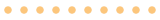Cloud Nets VideosJuly 19, 2022

# Season 2 Ep 2: Optics 101

### Optics 101 for Network Engineers

Let’s talk about optical modulation and some basic features of optics that us networking guys need to know.

Listen on your favorite podcast platform

Listen on Apple Podcasts
Listen on Spotify

Full Transcript

Hi and welcome back to CloudNets, where networks meet cloud.
Today we’re going to talk about optics again, and no not Run.
I will it’s okay.
I will take you today.
I know.
Yeah, let’s try it to do it alone.
Okay, so today we’re going to talk about optical modulation and some basic features of optics we the networking guys need to know when we talk to the optical guys.
So when we talk about optics, we talk about light and light as we know is a wave.
What’s that?
I know I know light is also a particle but we’re going to talk about light as a wave today.
Okay.
So light is a wave and as any wave it has a wavelength which is basically the the speed of light divided by the frequency of the light and the wavelength in all the communication run from 800 nanometers to 1600 nanometers.
And and it’s important to know because we need to know for an optical device which wavelength it works on.
So other than the wavelength we need to put digital information on top of the wave.
So how do we turn the sinus wave into bits ones and zero.
There are different ways to do it.
The most basic way is NRZ now return to zero in which we take one as a wave and zero as non wave.
So it’s simple that the length or the period of time in which we transmit a bit is either a science wave or nothing.
There are also more sophisticated manners like PAM4 in which we also use the amplitude of the wave, the height of the sinus in order to deliver bits.
So there are four heights.
There are four levels of wave and each level divides each level, describes two bits.
So it’s either 00, 01, 10, 11.
And we have four levels and two bits per symbol.
Again, symbol is the time in which we transmit a single wave but when it comes to zero and zero plus we talk about a much more sophisticated optical system.
First we use different wavelengths DWT and dense wavelength division multiplexing inside the model.
So it’s not only a single wave we transmit its multiple waves and each wave use a different wavelength because the light can travel throughout a single fiber in different wavelengths without each wavelength
interfering with the other.
So we get a modification of the data as the number of wavelengths over lambdas we put into the fiber.
So this is the WDM.
Another thing we do in and there are positives coherent optics.
That means we go from PAM4 or NRZ to QAM.
QAM is Quadrature Amplitude Modulation in which we use not only the amplitude of the wave but also the phase, the phase is this shift from the original sinus wave.
We can shift it to the left or to the right in a certain degree that is measured in degrees 0 to 360 in which we return to the same wave.
And if we put the aptitude and the phase, the angle on the graph and say the amplitude is the distance from the zero axis and the phase is the angle from the X axis.
We can paint or we can draw certain symbols.
Each symbols represents a certain amplitude at a certain angle or phase.
And in QAM 16 for instance we put 16 dots or 16 symbols over there.
And those 16 symbols represent 4 bits.
So each symbol, each period of time in which we transfer given information, package transfers 4 bits not 2 bits
like in PAM4 and not a single bit like in NRZ, more than that we use 2 polarizations.
So wave length or a light signal can go like this or it can go like this, figure of speech.
This doubles the amount of capacity we can put in a light.
This is the authorization.
So we use QAM 16 DP for polarization and we can transfer 8 bits per symbol.
So if our symbol rate for instance, for instance is 50 giga symbol per second.
So we can that means that in QAM 16 DP we can transfer 400 gigabit per second which is a lot for this symbol.
Right?
And this is what’s new in ZR and ZR-plus.
So what did we have today?